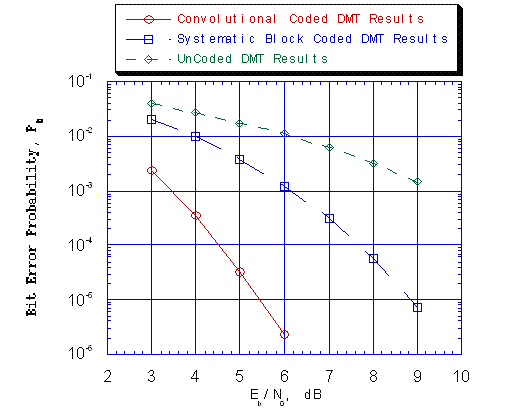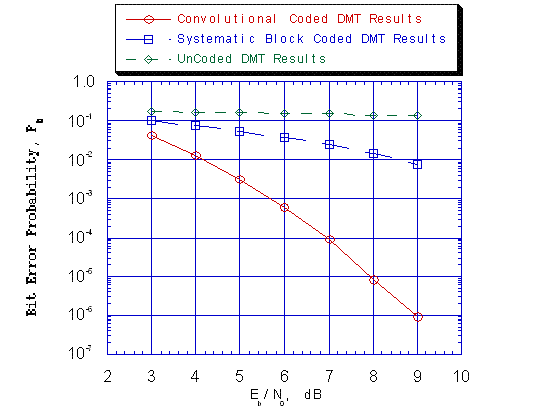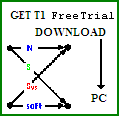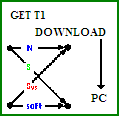# DMT Signaling Reference and AdvDCSM Application Example

Last Update: March 4, 2010, October 16, 2011.

The Figure 2 plot (shown below) displays the Simulated Bit Error Rate (BER) results for Convolutional Coded, Block Coded, and UnCoded cases where simulated Discrete MultiTone (DMT) Modulation Signaling occurs over a Non-Distorting, UnRestricted Bandwidth MultiCarrier/MultiChannel (MC).

If one compares Figure 1 (shown below or on the 'Nolta Simulated Systems Introduction' webpage) to Figure 2, one can readily see the effects of a bandwidth-limited MC on BER Performance as follows:

1) For UnCoded DMT, increasing the Eb/N0 has little effect in decreasing BER;

2) At low Eb/N0 and for UnCoded and Coded DMT, there is a order of magnitude difference in BER, i.e., loss in BER Performance; and

3) At high Eb/N0 and for UnCoded and Coded DMT, the BER spread increases dramatically.```Figure 2. Bit Error Probability for Coded and UnCoded DMT Signaling over
Non-Distorting, UnRestricted Bandwidth MultiCarrier/MultiChannel (MC)
with Additive White Gaussian Noise (AWGN): ```
```          r = 1/6, K = 4, [63,51,60,63] Best Non-Recursive Convolutional Code
and Viterbi Algorithm Decoder;```
```          L = 3, N = 6, [3,5,6] Systematic Block Code and Likelihood Block
Decoder;```
```          3-MC, {l} = [2,2,2]: Gray Coded QPSK, 4-PAM and 4-QAM,
SNRb: Eb/N0, QPSK = Eb/N0, 4-PAM = Eb/N0, 4-QAM;```
```          Non-Distorting, UnRestricted Bandwidth MC.
``````Figure 1. Bit Error Probability for Coded and UnCoded DMT Signaling over
Distorting Bandwidth-Constrained MultiCarrier/MultiChannel (MC)
with Additive White Gaussian Noise (AWGN): ```
```          r = 1/6, K = 4, [63,51,60,63] Best Non-Recursive Convolutional Code
and Viterbi Algorithm Decoder;```
```          L = 3, N = 6, [3,5,6] Systematic Block Code and Likelihood Block
Decoder;```
```          3-MC, {l} = [2,2,2]: Gray Coded QPSK, 4-PAM and 4-QAM,
SNRb: Eb/N0, QPSK = Eb/N0, 4-PAM = Eb/N0, 4-QAM;```
```          First Order Polynomial-based Low Pass Linear Filter MC.
```

Digital Communication Systems (Convolutional Coded, Block Coded, or UnCoded) using Discrete MultiTone (DMT) Modulation Signaling are complex systems that involve complex System and Subsystem models.

The AdvDCSM System Tool T1 is an invaluable computer-based software tool that can be readily used to model and simulate these DMT Systems for Non-Distorting, UnRestricted Bandwidth or Bandwidth-limited MultiCarrier/MultiChannel Channels.

T1 is NOW AVAILABLE as a TRIAL VERSION that will allow you to use T1, a Digital Communication (Information-Theoretic) System Modeling and Simulation software tool, for a Limited Period without Charge and to learn about the valuable utility of T1 in the study, evaluation, and possibly design of certain Complex Digital Communication Systems first hand before purchase.

```GET FREE TRIAL USE of T1, AdvDCSMT1DCSS by DOWNLOAD.
```
```BUY T1 (ADVDCSMT1DCSS software system tool)NOW.